# What is the Rossby number?

The Rossby number is used to describe whether a phenomenon is large-scale, i.e. if it is affected by earth’s rotation. But do we actually quantify if a fluid flow is affected by earth’s rotation?

Consider two quantities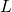and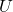, withbeing a characteristic scale-length of the phenomenon (ex. distance between two peaks, distance between two isobars, length of simulation domain) andthe horizontal velocity scale of the motion. The ratio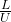is the time it takes to the motion to cover a distancewith velocity. If this time is bigger than the period of earth’s rotation, then the phenomenon IS affected by the rotation.

So if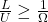, then the phenomenon IS a large-scale one. Thus we can define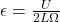and say that for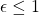a phenomenon is large scale. Phenomena with small Rossby number are dominated by Coriolis force behavior, while those with large Rossby number are dominated by inertial forces (ex: a tornado). However, rotational effects are more evident for low latitudes (i.e. near the equator), so the Rossby number can be different depending on where on earth we are.

(Notice that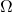is in theory equal to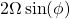, withbeing the earth rotational velocity and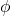the angle between the axis of rotation and the direction of fluid movement. In the geophysical context, flows are mostly horizontal (also due to density stratification in both atmosphere and ocean), so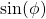can be approximated with 1. There is a bunch of different notation, but thisis also referred to as, called the Coriolis frequency.)